## Probability

 Question 1

If a random variable X has a Poisson distribution with mean 5, then the expectation E[(X + 2)2] equals _________.

 A 54 B 55 C 56 D 57
Engineering-Mathematics       Probability       GATE 2017 [Set-2]       Video-Explanation
Question 1 Explanation:
In Poisson distribution:
Mean = Variance
E(X) = E(X2) - (E(X))2 = 5
E(X2) = 5 + (E(X))2 = 5 + 25 = 30
So, E[(X + 2)2] = E[X2 + 4 + 4X]
= E(X2) + 4 + 4E(X)
= 30 + 4 + 4 × 5
= 54
 Question 2

Suppose Y is distributed uniformly in the open interval (1,6). The probability that the polynomial 3x2 + 6xY + 3Y + 6 has only real roots is (rounded off to 1 decimal place) _____.

 A 0.3 B 0.9 C 0.1 D 0.8
Engineering-Mathematics       Probability       GATE 2019       Video-Explanation
Question 2 Explanation:
Given polynomial equation is
3x2 + 6xY + 3Y + 6
= 3x2 + (6Y)x + (3Y + 6)
which is in the form: ax2 + bx + c
For real roots: b2 - 4ac ≥ 0
⇒ (6Y)2 - 4(3)(3Y + 6) ≥ 0
⇒ 36Y2 - 36Y - 72 ≥ 0
⇒ Y2 - Y - 2 ≥ 0
⇒ (Y+1)(Y-2) ≥ 0
Y = -1 (or) 2
The given interval is (1,6).
So, we need to consider the range (2,6).
The probability = (1/(6-1)) * (6-2) = 1/5 * 4 = 0.8
 Question 3

Two people, P and Q, decide to independently roll two identical dice, each with 6 faces, numbered 1 to 6. The person with the lower number wins. In case of a tie, they roll the dice repeatedly until there is no tie. Define a trial as a throw of the dice by P and Q. Assume that all 6 numbers on each dice are equi-probable and that all trials are independent. The probability (rounded to 3 decimal places) that one of them wins on the third trial is __________.

 A 0.021 B 0.022 C 0.023 D 0.024
Engineering-Mathematics       Probability       GATE 2018       Video-Explanation
Question 3 Explanation:
When two identical dice are rolled
⇾ A person wins who gets lower number compared to other person.
⇾ There could be “tie”, if they get same number.
Favorable cases = {(1, 1), (2, 2), (3, 3), (4, 4), (5, 5), (6, 6)}
Probability (tie) = 6/36 (when two dice are thrown, sample space = 6 × 6 = 36)
= 1/6
“Find the probability that one of them wins in the third attempt"
⇾ Which means, first & second time it should be tie and third time it should not be tie
⇾ P (tie) * P (tie) * P (not tie)
⇒ 1/6* 1/6 * (1 - 1/6)
⇒ (5/36×6)
= 0.138/6
= 0.023
 Question 4

Let X be a Gaussian random variable with mean 0 and variance σ2. Let Y = max(X, 0) where max(a,b) is the maximum of a and b. The median of Y is __________.

 A 0 B 1 C 2 D 3
Engineering-Mathematics       Probability       GATE 2017 [Set-1]       Video-Explanation
Question 4 Explanation:
Given that, Y = max(X,0),which means, Y is 0 for negative values of X and Y is X for positive values of X.
Median is a point, where the probability of getting less than median is 1/2 and probability of getting greater than median is 1/2.
From the given details, we can simply conclude that, median is 0. (0 lies exactly between positive and negative values)Question 5

P and Q are considering to apply for job. The probability that p applies for job is 1/4. The probability that P applies for job given that Q applies for the job 1/2 and The probability that Q applies for job given that P applies for the job 1/3.The probability that P does not apply for job given that Q does not apply for the job

 A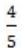BC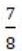DEngineering-Mathematics       Probability       GATE 2017 [Set-2]       Video-Explanation
Question 5 Explanation:
Probability that ‘P’ applies for a job = 1/4 = P(p) ⇾ (1)
Probability that ‘P’ applies for the job given that Q applies for the job = P(p/q) = 1/2 ⇾ (2)
Probability that ‘Q’ applies for the job, given that ‘P’ applies for the job = P(p/q) = 1/3 ⇾ (3)
Bayes Theorem:
(P(A/B) = (P(B/A)∙P(A))/P(B) ; P(A/B) = P(A∩B)/P(B))
⇒ P(p/q) = (P(q/p)∙P(p))/p(q)
⇒ 1/2 = (1/3×1/4)/p(q)
p(q) = 1/12×2 = 1/(6) (P(q) = 1/6) ⇾ (4)
From Bayes,
P(p/q) = (P(p∩q))/(P(q))
1/2 = P(p∩q)/(1⁄6)
(p(p∩q) = 1/12)
We need to find out the “probability that ‘P’ does not apply for the job given that q does not apply for the job = P(p'/q')
From Bayes theorem,
P(p'/q') = (P(p'∩q'))/P(q') ⇾ (5)
We know,
p(A∩B) = P(A) + P(B) - P(A∪B)
also (P(A'∩B') = 1 - P(A∪B))
P(p'∩q') = 1 - P(p∪q)
= 1 - (P(p) + P(q) - P(p∩q))
= 1 - (P(p) + P(q) - P(p) ∙ P(q))
= 1 - (1/4 + 1/6 - 1/12)
= 1 - (10/24 - 2/24)
= 1 - (8/24)
= 2/3
(P(p'∩q') = 2/3) ⇾ (6)
Substitute in (5),
P(p'⁄q') = (2⁄3)/(1-P(q)) = (2⁄3)/(1-1/6) = (2⁄3)/(5⁄6) = 4/5
(P(p'/q') = 4/5)
 Question 6

For any discrete random variable X, with probability mass function P(X=j)=pj, pj≥0, j∈{0, ..., N} and, define the polynomial function. For a certain discrete random variable Y, there exists a scalar β∈[0,1] such that gy(Z)=(1-β+βz)N. The expectation of Y is

 A Nβ(1 - β) B Nβ C N(1 - β) D Not expressible in terms of N and β alone
Engineering-Mathematics       Probability       GATE 2017 [Set-2]       Video-Explanation
Question 6 Explanation:
For a discrete random variable X,
Given gy (z) = (1 - β + βz)N ⇾ it is a binomial distribution like (x+y)n
Expectation (i.e., mean) of a binomial distribution will be np.
The polynomial function,
givenMean of Binomial distribution of b(xj,n,p)=The probability Mass function,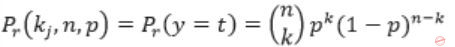Given:Question 7

A probability density function on the interval [a,1] is given by 1/x2 and outside this interval the value of the function is zero. The value of a is _________.

 A 0.7 B 0.6 C 0.5 D 0.8
Engineering-Mathematics       Probability       GATE 2016 [Set-1]       Video-Explanation
Question 7 Explanation:
The property of probability density function is area under curve = 1
or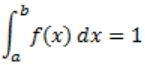where (a, b) is internal and f(x) is probability density function.
Given,
f(x) = 1/x2 , a≤x≤1
The area under curve,- 1 + 1/a = 1
1/a = 2
a = 0.5
 Question 8
Consider the following experiment.
Step1. Flip a fair coin twice.
Step2. If the outcomes are (TAILS, HEADS) then output Y and stop.
Step4. If the outcomes are (TAILS, TAILS), then go to Step 1.
The probability that the output of the experiment is Y is (up to two decimal places) ________.
 A 0.33 B 0.34 C 0.35 D 0.36
Engineering-Mathematics       Probability       GATE 2016 [Set-1]       Video-Explanation
Question 8 Explanation:
If a coin is flipped twice, the possible outcomes {HH, HT, TH, TT}
Stop conditions:
If outcome = TH then Stop [output 4] --------------- (1)
else
outcome = HH/ HT then Stop [output N] -------------- (2)
We get ‘y’ when we have (1) i.e., ‘TH’ is output.
(1) can be preceded by ‘TT’ also, as ‘TT’ will reset (1) again
Probability of getting y = TH + (TT)(TH) + (TT)(TT)(TH) + …
= 1/2 × 1/2 + 1/2 × 1/2 × 1/2 × 1/2 + ...
= (1/4)/(1-1/4)
= 1/3
= 0.33
 Question 9

Suppose that a shop has an equal number of LED bulbs of two different types. The probability of an LED bulb lasting more than 100 hours given that it is of Type 1 is 0.7, and given that it is of Type 2 is 0.4. The probability that an LED bulb chosen uniformly at random lasts more than 100 hours is _________.

 A 0.55 B 0.56 C 0.57 D 0.58
Engineering-Mathematics       Probability       GATE 2016 [Set-2]       Video-Explanation
Question 9 Explanation:The bulbs of Type 1, Type 2 are same in number.
So, the probability to choose a type is 1/2.
The probability to choose quadrant ‘A’ in diagram is
P(last more than 100 hours/ type1) = 1/2 × 0.7
P(last more than 100 hours/ type2) = 1/2 × 0.4
Total probability = 1/2 × 0.7 + 1/2 × 0.4 = 0.55
 Question 10

Suppose Xi for i = 1, 2, 3 are independent and identically distributed random variables whose probability mass functions are Pr[Xi = 0] = Pr[Xi = 1]=1/2 for i = 1, 2, 3. Define another random variable Y = X1X2 ⊕ X3, where ⊕ denotes XOR. Then Pr[Y = 0|X3 = 0] = _______________.

 A 0.75 B 0.76 C 0.77 D 0.78
Database-Management-System       Probability       GATE 2015 [Set-3]
Question 10 Explanation: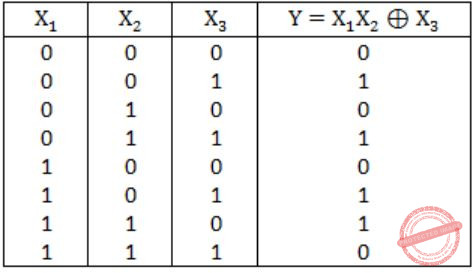Pr(Y=0/ X3=0) = Pr(Y=0 ∩ X3=0)/ Pr(X3=0)
= 3/8 / 4/8 = 3/4 = 0.75
 Question 11

Four fair six-sided dice are rolled. The probability that the sum of the results being 22 is X/1296. The value of X is ___________.

 A 10 B 11 C 12 D 13
Engineering-Mathematics       Probability       GATE 2014 [Set-1]
Question 11 Explanation:
Each dice can result from {1, 2, 3, 4, 5, 6}
To get ‘22’ as Sum of four outcomes
x1 + x2 + x3 + x4 = 22
The maximum Sum = 6+6+6+6 = 24 which is near to 22
So, keeping three 6’s, 6+6+6+x = 22
x = 4 combination① = 6 6 6 4
Keeping two 6’s, 6+6+x1+x2 = 22
x1+x2 = 10 possible x’s (5, 5) only
combination② = 6 6 5 5
No. of permutation with 6664 = 4!/ 3! = 4
“ “ “ 6655 = 4!/ 2!2! = 6
Total = 4+6 = 10 ways out of 6×6×6×6 = 1296
Pnb (22) = 10/1296 ⇒ x = 10
 Question 12

The security system at an IT office is composed of 10 computers of which exactly four are working. To check whether the system is functional, the officials inspect four of the computers picked at random (without replacement). The system is deemed functional if at least three of the four computers inspected are working.  Let the probability that the system is deemed functional be denoted by p. Then 100p =_____________.

 A 11.9 B 11.91 C 11.92 D 11.93
Engineering-Mathematics       Probability       GATE 2014 [Set-2]
Question 12 Explanation:
There are 10 systems.
Out of which ‘4’ are chosen at random without replaced and created.
If at least three of them are working then system is deemed functional
i.e., there should be only ‘one’ non-working system in set of ‘4’.
It is possible with combination
W – W – W – N,
W – W – N – W,
W – N – W – W,
N – W – W – W.
For W – W – W – N, the probability = (choosing working out of 10) × (choosing working out of 9) × (choosing working out of 8) × (choosing non-working out of 7)
= 4/10×3/9×2/8×1/7
where 4/10 ⇒ 4 working out of 10
3/9 ⇒ 3 working are remaining out of ‘9’ as ‘1’ is already taken
For ‘4’ Sum combinations
Total probability = 4×[4/10×3/9×2/8×1/7] = 600/5040
We need 100p ⇒ 100×600/5040 = 11.90
 Question 13

Each of the nine words in the sentence "The quick brown fox jumps over the lazy dog" is written on a separate piece of paper. These nine pieces of paper are kept in a box. One of the pieces is drawn at random from the box. The expected length of the word drawn is _____________. (The answer should be rounded to one decimal place.)

 A 3.9 B 4 C 4.1 D 4.2
Engineering-Mathematics       Probability       GATE 2014 [Set-2]
Question 13 Explanation:
"The quick brown fox jumps over the lazy dog"
There are ‘9’ words in this sentence.
No. of characters in each word
The (3)
quick (5)
brown (5)
fox (3)
jumps (5)
over (4)
the (3)
lazy (4)
dog (3)
Each word has equal probability.
So expected length = 3×1/9+5×1/9+5×1/9+3×1/9+5×1/9+ 4×1/9+3×1/9+4×1/9+3×1/9
= 35/9
= 3.9
 Question 14

The probability that a given positive integer lying between 1 and 100 (both inclusive) is NOT divisible by 2, 3 or 5 is ______.

 A 0.259 to 0.261 B 0.260 to 0.262 C 0.261 to 0.263 D 0.262 to 0.264
Engineering-Mathematics       Probability       GATE 2014 [Set-2]
Question 14 Explanation:
Let A = divisible by 2, B = divisible by 3 and C = divisible by 5, then
n(A)=50, n(B)=33, n(C)=20
n(A∩B)=16, n(B∩C)=6, n(A∩C)=10
n(A∩B∩C)=3
P(A∪B∪C) = P(A)+P(B)+P(C)-P(A∩B)-P(B∩C) -P(A∩C)+P(A∩B∩C) = 74/100
∴ Required probability is P(A∩B∩C) = 1-P(A∪B∪C) = 0.26
 Question 15

Let S be a sample space and two mutually exclusive events A and B be such that A∪B = S. If P(∙) denotes the probability of the event, the maximum value of P(A)P(B) is __________.

 A 0.25 B 0.26 C 0.27 D 0.28
Engineering-Mathematics       Probability       GATE 2014 [Set-3]
Question 15 Explanation:
We know that
P(A∪B) = P(A) + P(B) + P(A∩B) = 1 →①
But, as A and B are mutually exclusive events
P(A∩B) = 0
∴ P(A∪B) = P(A) + P(B) = 1 →②
Arithmetic mean of two numbers ≥ Geometric mean of those two numbers
(P(A)+P(B))/2 ≥ √(P(A)∙P(B))
1/2 ≥ √(P(A)∙P(B)) (∵from ②)
Squaring on both sides
1/4 ≥ P(A)∙P(B)
P(A)∙P(B) ≤ 1/4
∴ Maximum value of P(A)P(B) = 1/4 = 0.25
 Question 16

Suppose p is the number of cars per minute passing through a certain road junction between 5 PM and 6 PM, and p has a Poisson distribution with mean 3. What is the probability of observing fewer than 3 cars during any given minute in this interval?

 A 8/(2e3) B 9/(2e3) C 17/(2e3) D 26/(2e3)
Engineering-Mathematics       Probability       GATE 2013
Question 16 Explanation:
The formula for the Poisson probability mean function
P(x : λ) = (e λx)/x! for x = 0,1,2….
‘λ’ is the average number (mean)
Given that mean = λ = 3
The probability of observing fewer than three cars is
P(zero car) + P(one car) + P(two cars)
= (e-3 30)/0!+(e-3 31)/1!+(e-3 32)/2!
= e-3+e-3∙3+(e-3)∙9)/2
= (17e-3<)/2
= 17/(2e3 )
 Question 17

Consider a random variable X that takes values +1 and −1 with probability 0.5 each. The values of the cumulative distribution function F(x) at x = −1 and +1 are

 A 0 and 0.5 B 0 and 1 C 0.5 and 1 D 0.25 and 0.75
Instruction-Pipelining       Probability       GATE 2012
Question 17 Explanation:
Cumulative probability is sum of probabilities of all other points till the given value.
The sum of probabilities at x=1, x=-1 itself is 0.5+0.5 = 1. It is evident that, there is no probability for any other values.
The F(x=-1) is 0.5 as per given probabilities and
F(x=1) = sum of F(x=-1) +F(x=0) = ...f(X=1) = 0.5 +0.5 = 1
 Question 18

Suppose a fair six-sided die is rolled once. If the value on the die is 1, 2, or 3, the die is rolled a second time. What is the probability that the sum total of values that turn up is at least 6?

 A 10/21 B 5/12 C 2/3 D 1/6
Engineering-Mathematics       Probability       GATE 2012
Question 18 Explanation:
The value on the die for first time rolling = {1, 2, 3}
The value on second time can be {1, 2, 3, 4, 5, 6}
So the Sum can beWe have Sample space = 36
The no. of events where (Sum = atleast 6) = {6, 7, 6, 7, 8, 6, 7, 8, 9}
So the probability atleast ‘6’ while getting {1, 2, 3} in first time = 9/36 → ①
If we get ‘6’ in the first time itself, then we do not go for rolling die again.
So, its probability = 1/6
Total probability = 1/6 + 9/36 = 1/6 + 1/4 = 10/24 = 5/12
 Question 19

If the difference between the expectation of the square of a random variable (E[X2]) and the square of the expectation of the random variable (E[X])2 is denoted by R, then

 A R = 0 B R < 0 C R ≥ 0 D R > 0
Engineering-Mathematics       Probability       GATE 2011
Question 19 Explanation:
We know that difference of E(X2) and E(X))2 is nothing but variance or V(X) which is always greater than or equal to zero.
So the answer will be R≥0.
 Question 20

If two fair coins are flipped and at least one of the outcomes is known to be a head, what is the probability that both outcomes are heads?

 A 1/3 B 1/4 C 1/2 D 2/3
Engineering-Mathematics        Probability       GATE 2011
Question 20 Explanation:
Sample space = {HH, HT, TH}
Required probability = 1/3
 Question 21

A deck of 5 cards (each carrying a distinct number from 1 to 5) is shuffled thoroughly. Two cards are then removed one at a time from the deck. What is the probability that the two cards are selected with the number on the first card being one higher than the number on the second.

 A 1/5 B 4/25 C 1/4 D 2/5
Engineering-Mathematics       Probability       GATE 2011
Question 21 Explanation:
The possible events are
(2,1) (3,2) (4,3) (5,4).
So only 4 possibilities are there and sample space will be,
5C1 × 4C1 = 20
So probability = 4/20 = 1/5
 Question 22

Consider a company that assembles computers. The probability of a faulty assembly of any computer is p. The company therefore subjects each computer to a testing process. This testing process gives the correct result for any computer with a probability of q. What is the probability of a computer being declared faulty?

 A pq + (1 - p)(1 - q) B (1 - q)p C (1 - p)q D pq
Engineering-Mathematics       Probability       GATE 2010
Question 22 Explanation:
The probability of the computer being declared faulty is,
= Probability of testing process gives the correct result × Probability that computer is faulty + Probability of testing process giving incorrect result × Probability that computer is not faulty
= p × q + (1 - p) (1 - q)
 Question 23

What is the probability that divisor of 1099 is a multiple of 1096?

 A 1/625 B 4/625 C 12/625 D 16/625
Engineering-Mathematics       Probability       GATE 2010
Question 23 Explanation:
Probability that divisor of 1099 is a multiple of 1096
We can write 1099 as 1096×103
So, (1099)/(1096) to be a whole number, [1096×103/1096] ➝ (1)
We can observe that every divisor of 103 is a multiple of 1096
So number of divisor of 103 to be found first
⇒ 103 = (5×2)3 = 23×53
No. of divisors = (3 + 1) (3 + 1) = 16
Total number of divisor of 1099 are 1099 = 299×599 = 100×100 = 10000
Probability that divisor of 1099 is a multiple of 1096 is
⇒ 16/10,000
 Question 24

An unbalanced dice (with 6 faces, numbered from 1 to 6) is thrown. The probability that the face value is odd is 90% of the probability that the face value is even. The probability of getting any even numbered face is the

If the probability that the face is even given that it is greater than 3 is 0.75, which one of the following options is closest to the probability that the face value exceeds 3?

 A 0.453 B 0.468 C 0.485 D 0.492
Engineering-Mathematics       Probability       GATE 2009
Question 24 Explanation:
P(0) = Probability of getting odd no. face.
P(e) = Probability of getting even no. face.
It is given that,
P(0) = 0.9 P(e) ----- (I)
Also we know that,
P(0) + P(e) = 1 ----- (II)
Solving equation (I) and (II) we get,
P(e) = 0.52
Also even no. can be 2 or 4 or 6.
And given in question that P(2) = P(4) = P(6).
So, 3 × P(2) = 0.52
P(2) = 0.175
So, P(2) = P(4) = P(6) = 0.175
Also in question it is given that,
P(e/>3) = 0.75
P(even no. greater than 3)/ P(no. greater than 3) = 0.75
P(4,6)/P(>3) = 0.75
(0.175×2)/P(>3) = 0.75
P(>3) = 0.35/0.75 = 0.467
 Question 25

Aishwarya studies either computer science or mathematics everyday. If she studies computer science on a day, then the probability that she studies mathematics the next day is 0.6. If she studies mathematics on a day, then the probability that she studies computer science the next day is 0.4. Given that Aishwarya studies computer science on Monday, what is the probability that she studies computer science on Wednesday?

 A 0.24 B 0.36 C 0.4 D 0.6
Engineering-Mathematics       Probability       GATE 2008
Question 25 Explanation:
→ Aishwarya studied CS on Monday. Then we have two possibilities to she study computer science on wednesday.
(i) She study Mathematics on Tuesday and computer science on wednesday.
⇒ 0.6×0.4
⇒ 0.24
(ii) She study computer science on Tuesday and computer science on wednesday.
⇒ 0.4×0.4
⇒ 0.16
→ The probability that she study computer science on wednesday is
0.24+0.16 = 0.40
 Question 26

Let X be a random variable following normal distribution with mean +1 and variance 4. Let Y be another normal variable with mean -1 and variance unknown. If P(X ≤ -1) = P(Y ≥ 2), the standard deviation of Y is

 A 3 B 2 C √2 D 1
Engineering-Mathematics       Probability       GATE 2008
Question 26 Explanation:
P(X ≤ -1) = P(Y ≥ 2)
We can compare their values using standard normal distributions.The above equation satisfies when σy will be equal to 3.
 Question 27

Suppose we uniformly and randomly select a permutation from the 20! Permutations of 1, 2, 3,….., 20. What is the probability that 2 appears at an earlier position than any other even number in the selected permutation?

 A 1/2 B 1/10 C 9!/20! D None of these
Engineering-Mathematics       Probability       GATE 2007
Question 27 Explanation:
1, 2, 3, 4, …….20
→ Total no. of possible even number = 10
→ Here we are not considering odd number.
→ The probability that 2 appears at an earlier position than any other even number is =1/10
 Question 28

We are given a set X = {x1, .... xn} where xi = 2i. A sample S ⊆ X is drawn by selecting each xi independently with probability pi = 1/2. The expected value of the smallest number in sample S is:

 A 1/n B 2 C √n D n
Engineering-Mathematics       Probability       GATE 2006
Question 28 Explanation:
The smallest element in sample S would be xi for which i is the smallest.
The given probability Pi is for selection of each item independently with probability 1/2.
Now, Probability for x1 to be smallest in S = 1/2
Now, Probability for x2 to be smallest in S = Probability of x1 not being in S × Probability of x2 being in S
= 1/2 × 1/2
Similarly, Probability xi to be smallest = (1/2)i
Now the Expected value isQuestion 29

For each element in a set of size 2n, an unbiased coin is tossed. The 2n coin tosses are independent. An element is chosen if the corresponding coin toss were head. The probability that exactly n elements are chosen is:

 ABCD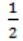Engineering-Mathematics       Probability       GATE 2006
Question 29 Explanation:
Total number of coins = 2n
No. of elements selected = n
Probability of getting head = ½
Probability of n heads out of 2n coin tosses is
2nCn*(1/2)n*(1/2)n = 2nCn/4n
 Question 30

Let f(x) be the continuous probability density function of a random variable X. The probability that a < X ≤ b, is:

 A f(b - a) B f(b) - f(a) CDEngineering-Mathematics       Probability       GATE 2005
Question 30 Explanation:
f(x) be the continuous probability density function of random variable X.
Then the probablity be area of the corresponding curve i.e.,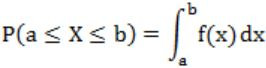Question 31

Box P has 2 red balls and 3 blue balls and box Q has 3 red balls and 1 blue ball. A ball is selected as follows:

```(i) Select a box
(ii) Choose a ball from the selected box such that each ball in
the box is equally likely to be chosen. The probabilities of
selecting boxes P and Q are (1/3) and (2/3), respectively.```

Given that a ball selected in the above process is a red ball, the probability that it came from the box P is

 A 4/19 B 5/19 C 2/9 D 19/30
Engineering-Mathematics       Probability       GATE 2005
Question 31 Explanation:
P → 2 red, 3 blue
Q → 3 red, 1 blue
The probability of selecting a red ball is
(1/3)(2/5) + (2/3)(3/4)
2/15 + 1/2 = 19/30
The probability of selecting a red ball from P
(1/3) * (2/5) = 2/15
→ The colour of ball is selected is to be red and that is taken from the box P.
⇒ Probability of selecting a red ball from P/Probability of selecting a red ball
⇒ (2/15)/(19/30)
⇒ 4/19
 Question 32

A random bit string of length n is constructed by tossing a fair coin n times and setting a bit to 0 or 1 depending on outcomes head and tail, respectively. The probability that two such randomly generated strings are not identical is:

 A 1/2n B 1 - 1/n C 1/n! D 1-(1/2n)
Engineering-Mathematics       Probability       GATE 2005
Question 32 Explanation:
Total combinations of strings that can be generated are 2n. We will get one such string in the first experiment. So, favourable cases for the second string are 2n - 1, so that it doesn't match with the previous generated string.
Hence Probability = (2n - 1) /2n = 1 - 1/2n
 Question 33

If a fair coin is tossed four times. What is the probability that two heads and two tails will result?

 A 3/8 B 1/2 C 5/8 D 3/4
Engineering-Mathematics       Probability       GATE 2004
Question 33 Explanation:
A fair coin is tossed 4 times.
Then total number of possibilities = 24 = 16
No. of possibilities getting 2 heads and 2 tails is
HHTT, HTHT, TTHH, THTH, THHT, HTTH = 6
Probability of getting 2 heads and 2 tails is
= No. of possibilities/Total no. of possibilities = 6/16 = 3/8
 Question 34

An examination paper has 150 multiple-choice questions of one mark each, with each question having four choices. Each incorrect answer fetches -0.25 mark. Suppose 1000 students choose all their answers randomly with uniform probability. The sum total of the expected marks obtained by all these students is:

 A 0 B 2550 C 7525 D 9375
Engineering-Mathematics       Probability       GATE 2004
Question 34 Explanation:
Probability of choosing a correct answer = 1/4
Probability of selecting a wrong answer = 3/4
Expected marks for each question = (1/4) × 1 + (3/4) -(0.25)
= 1/4 + (-3/16)
= 4-3/16
= 1/16
= 0.0625
Expected marks for 150 questions = 150 × 0.625 = 9.375
The sum total of expected marks obtained by 1000 students is = 1000×9.375 = 9375
 Question 35

Two n bit binary strings, S1 and S2, are chosen randomly with uniform probability. The probability that the Hamming distance between these strings (the number of bit positions where the two strings differ) is equal to d is

 A nCd /2n B nCd / 2d C d/2n D 1/2d
Engineering-Mathematics       Probability       GATE 2004
Question 35 Explanation:
n binary bits with difference 'd' then no. of favourable cases = nCd
Total no. of cases where n positions have any binary bit = 2n
The probability of 'd' bits differ = nCd / 2n
 Question 36

A point is randomly selected with uniform probability in the X-Y plane within the rectangle with corners at (0,0), (1,0), (1,2) and (0,2). If p is the length of the position vector of the point, the expected value of p2 is

 A 2/3 B 1 C 4/3 D 5/3
Engineering-Mathematics       Probability       GATE 2004
Question 36 Explanation:Above diagram shows the scenario of our question.
The length p of our position vector (x,y) is
p = √x2 + y2
p2 = x2 + y2
E(p2) = E(x2 + y2) = E(x2) + E(y2)
Now we need to calculate the probability density function of X and Y.
Since distribution is uniform,
X goes from 0 to 1, so PDF(x) = 1/1-0 = 1
Y goes from 0 to 2, so PDF(y) = 1/2-0 = 1/2
Now we evaluate,E(p2) = E(x2) + E(y2) = 5/3
There are 36 questions to complete.

Register Now